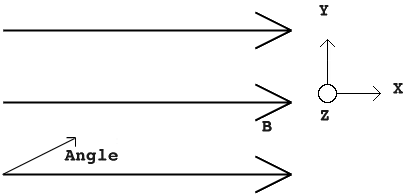# T or F: Magnetism and Magnetic Field

• choco_moo
In summary, when a 2 kg particle with a charge of 97 uC enters a uniform 7 T magnetic field at a speed of 26 m/s and an angle of 52 degrees, the work done by the field is zero, the y-component of the velocity is unchanged, the force on the particle is in the -z direction, the particle follows a spiral path, and the speed remains unchanged. The direction of the force can be determined using the right hand rule, and the particle's velocity is affected by the changing direction of the force.

## Homework Statement

A 2 kg particle carrying a charge of 97 uC enters a uniform 7 T magnetic field at a speed of 26 m/s and with an angle of 52o with respect to the field lines, as shown in the figure. Answer the following questions:A) The work done by the field on the particle is zero as the force is normal to the displacement.
B) The y-component of the particle's velocity is unchanged as it passes through the B-Field.
C) The Force on the particle is in the -z direction.
D) The particle follows a circular path as the force is normal to the velocity.
E) The particle's speed is unchanged as it passes through the B-Field.

## The Attempt at a Solution

A) The work done by the field on the particle is zero as the force is normal to the displacement.T but I'm not sure.
B) The y-component of the particle's velocity is unchanged as it passes through the B-Field.F because the field lines are perpendicular to the y-component. Parallel components remain unchanged.
C) The Force on the particle is in the -z direction.No idea
D) The particle follows a circular path as the force is normal to the velocity.No idea
E) The particle's speed is unchanged as it passes through the B-Field.T because B can only change the direction of the velocity.

Any help is much appreciated!

How do you find the direction of the force, if you know the direction of the field and the direction of the velocity of the particle? You need this for A and C

willem2 said:
How do you find the direction of the force, if you know the direction of the field and the direction of the velocity of the particle? You need this for A and C

I don't know. I assume I have to use the right hand rule, but I don't get how that works. The particle is moving through the field at 52 degrees, but work is zero if the displacement and force are perpendicular to each other. I don't see how work can be zero because isn't the displacement going to be 52 degrees on every line? I am so confused.

choco_moo said:
I don't know. I assume I have to use the right hand rule, but I don't get how that works. The particle is moving through the field at 52 degrees, but work is zero if the displacement and force are perpendicular to each other. I don't see how work can be zero because isn't the displacement going to be 52 degrees on every line? I am so confused.

Whatever the angle of the field and the velocity, it's always possible to have a
force that's perpendicular to both.

willem2 said:
Whatever the angle of the field and the velocity, it's always possible to have a
force that's perpendicular to both.
So I figured out the answers:
A) True, because of what you just stated.
B) False, because the direction is always changing. The B-field is constantly moving.
C) True, because of the right hand rule. -z is pointing into the page.
D) False, because the B-field is always moving, so the particle would follow a spiral-like shape instead of circular.
E) True, because if work is zero then speed can not change.

Thanks for the help!

The B field isn't moving
but the direction of the force on the particle is.

The particle follows a spiral and not a circle, because the x component of its velocity is in the direction of the B field and the force on the particle can't have a component in the x direction, so the x component of the velocity can't change.